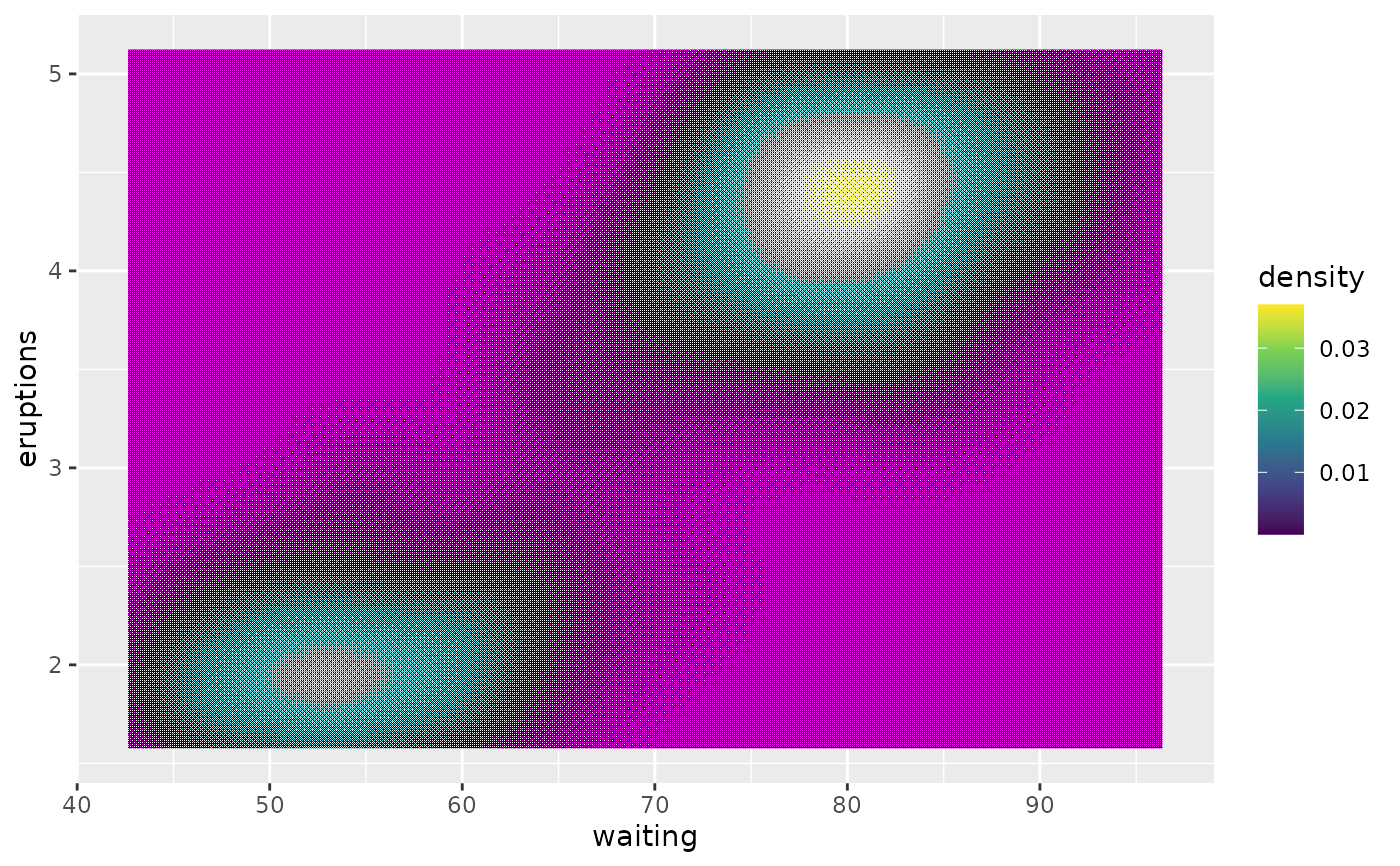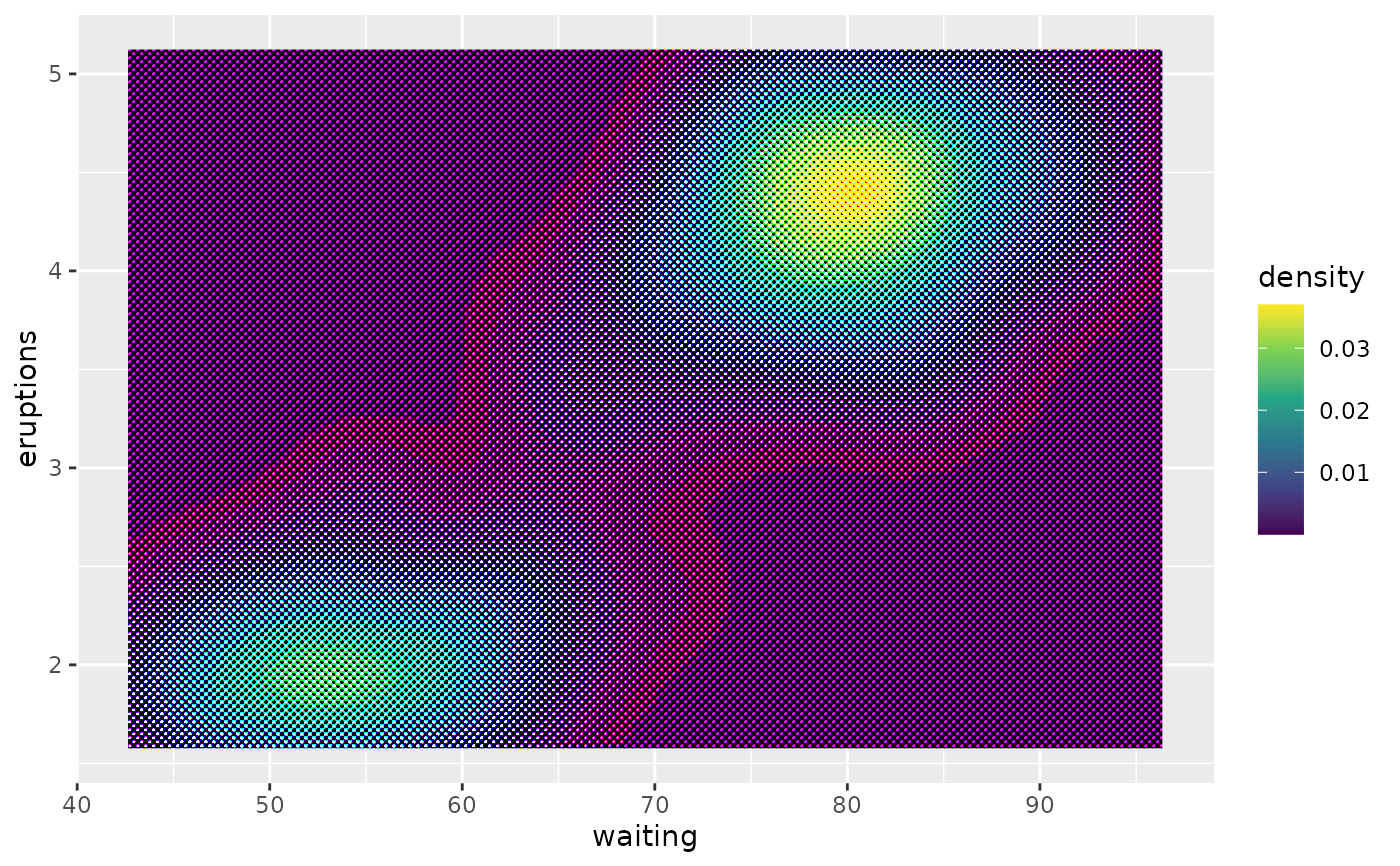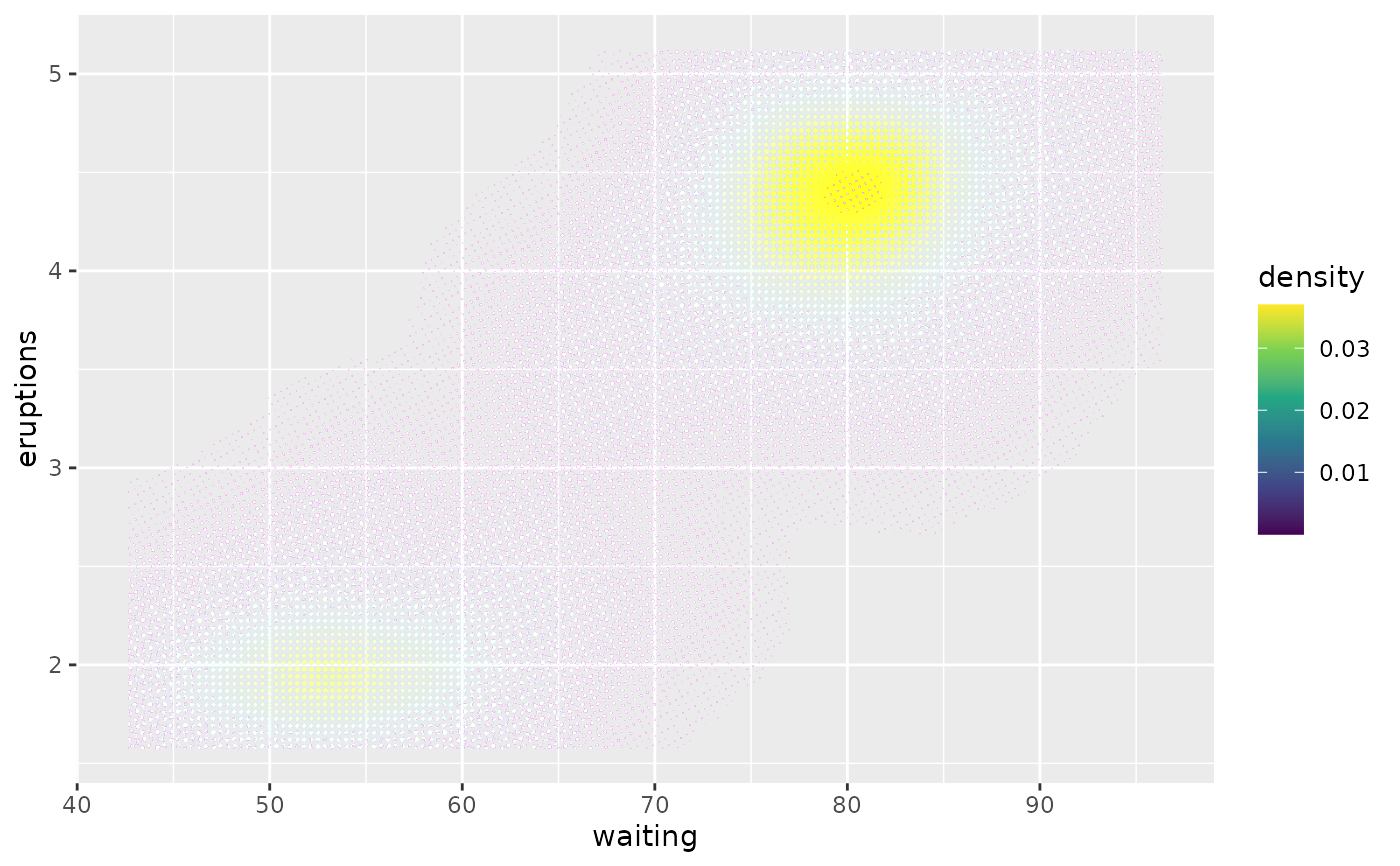These filters reduces the number of colours in your layer and uses various threshold maps along with a dithering algorithm to disperse colour error.

with_circle_dither(
x,
map_size = 7,
levels = NULL,
black = TRUE,
colourspace = "sRGB",
offset = NULL,
...
)

with_custom_dither(
x,
map = "checks",
levels = NULL,
colourspace = "sRGB",
offset = NULL,
...
)

with_halftone_dither(
x,
map_size = 8,
levels = NULL,
angled = TRUE,
colourspace = "sRGB",
offset = NULL,
...
)

with_ordered_dither(x, map_size = 8, levels = NULL, colourspace = "sRGB", ...)

## Arguments

x

A ggplot2 layer object, a ggplot, a grob, or a character string naming a filter

map_size

One of 2, 3, 4, or 8. Sets the threshold map used for dithering. The larger, the better approximation of the input colours

levels

The number of threshold levels in each channel. Either a single integer to set the same number of levels in each channel, or 3 values to set the levels individually for each colour channel

black

Should the map consist of dark circles expanding into the light, or the reverse

colourspace

In which colourspace should the dithering be calculated

offset

The angle offset between the colour channels

...

Arguments to be passed on to methods. See the documentation of supported object for a description of object specific arguments.

map

The name of the threshold map to use as understood by magick::image_ordered_dither()

angled

Should the halftone pattern be at an angle or orthogonal

## Value

Depending on the input, either a grob, Layer, list of Layers, guide, or element object. Assume the output can be used in the same context as the input.

Other dithering filters: with_dither()

## Examples

library(ggplot2)

# Ordered dither
ggplot(faithfuld, aes(waiting, eruptions)) +
with_ordered_dither(
geom_raster(aes(fill = density), interpolate = TRUE)
) +
scale_fill_continuous(type = 'viridis')# Halftone dither
ggplot(faithfuld, aes(waiting, eruptions)) +
with_halftone_dither(
geom_raster(aes(fill = density), interpolate = TRUE)
) +
scale_fill_continuous(type = 'viridis')# Circle dither with offset
ggplot(faithfuld, aes(waiting, eruptions)) +
with_circle_dither(
geom_raster(aes(fill = density), interpolate = TRUE),
offset = 29,
colourspace = 'cmyk'
) +
scale_fill_continuous(type = 'viridis')